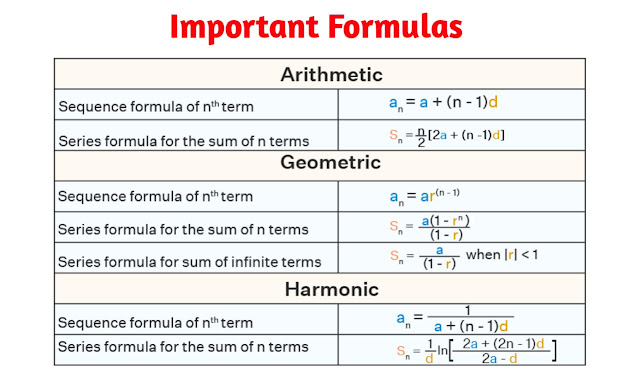# Sequence and Series Class 11 Mathematics Solutions | Exercise - 4.1## Chapter - 4  Sequence and Series

In this chapter, we are basically going to learn about the topics  related to sequence and series such as:
- Arithmetic Progression (AP)
- Geometric Progression (GP)
- Sum of Infinite Arithmetic Progression (SAP)
- Sum of Infinite Geometric Progression (SGP)
- Arithmetic Mean (AM)
- Geometric Mean (GM)
- Harmonic Mean (HM)

So before moving towards the solution section, let us have a little concept about the subtopics related to sequence and series as well as some important formulas related to this chapter.

### Introduction

The Sequence and Series chapter of Class 11 Mathematics is the study of patterns found in sequences and series of numbers. This chapter introduces the student to the concept of mathematical sequences and series, and how to work with them. It also covers topics such as arithmetic and geometric progressions, infinite series, and the summation of finite and infinite series.

The aim of this chapter is to equip the student with the knowledge and skills to identify, analyse and solve problems involving sequences and series. By the end of the chapter, the student should be able to determine the sum of a given sequence, find the nth term of a sequence, and be able to find the sum of a finite or infinite series. They should also be able to use the properties of arithmetic and geometric progressions to solve equations.

### Arithmetic Sequence

A sequence of numbers in which a certain number can be added to (or subtracted from) any term to get the next term is called an arithmetic sequence (or arithmetic progression). In other words, a sequence in which the difference between successive terms always has the same value is called an arithmetic sequence. The arithmetic sequence is also known as the arithmetic progression. As an abbreviation, the arithmetic sequence and the arithmetic progression are respectively written as A.S. and A.P. respectively. For examples,
(a) 1,3,5,7,9,...
(b) 9,-6,-3,0,3,...

### Geometric Sequences

A geometric sequence is a sequence of numbers in which the ratio of any term to its preceding term is always the same The geometric sequence is also known as the geometric progression. Geometric sequence and geometric progression are written as G.S., and G.P respectively. Examples of such sequences are;
(a) 1,2,4,8, 16,.....
(b) 3,-9,27,-81,.......

### Harmonic Sequences

A sequence is said to be a harmonic sequence if the reciprocals of its terms form an arithmetic sequence. The harmonic sequence is also known as the harmonic progression. Harmonic sequence and harmonic progression are also written as H.S. and H.P. respectively. Some examples of harmonic sequence (harmonic progression) are;
(a) 1,1/2,1/3, 1/4 ,......
(b) 1,1/3,1/5, 1/7 ,.....### Exercise - 4.1

This PDF contains only the solution of exercises - 4.1 of class 11 Sequence and Series chapter. If you are searching for other exercises then you can find them above. You just have to click on the respective buttons to view the required exercise solutions.

NoteScroll the PDF to view all Solution

You are not allowed to post this PDF in any website or social platform without permission.

## Is Class 11 Mathematics Guide Helpful For Student ?

This Notes is intended to help students who struggle with math problems. Student should not completely depend on this Notes for all exercises. If you rely on this Note and simply copy it as is, it may affect your study. Student should also use their own willpower and try to solve problems themselves.

You may use this mathematics guide PDF as a reference. Check all the answers before copying because all the answers may not be correct. There may be some minor mistakes in the Notes.

## How to secure good marks in Mathematics Exam?

In case you didn't know, I also am a student. Being a student isn't an easy task as you have to study many different subjects at the same time. From my perspective, most students are weak in mathematics. You can take me as an example as I am also weak in mathematics, and I face some difficulties when I try to answer mathematics questions.

To achieve good marks in mathematics, you must practice them every day. Once each week, you should review all the exercises that were taught in class. When you are solving math problems, start with easy questions that you already know. Doing so you won't get bored instead you'll start enjoying it.

I think all of us can agree that mathematics is more than just practising. It is important that you understand the basic concepts of the problem, especially in grade 11. Once you understand the main concepts of the problem, you can easily solve any problem where similar concepts are applied.

Whenever a teacher uses examples in order to explain a concept, all students tend to remember those examples, but it is a mistake to do so. If you give proper time and attention to your practice with proper technique, then you will definitely be able to score a good mark in your examination if you create your own formula that you won't forget later.

Disclaimer: The content on this site is for educational purposes only. If there is any content on this site that belongs to you, please contact us through the contact form.

If you have any queries then feel free to comment down.
No Comment